## Chapter 3 Pair of Linear Equations in Two Variables R.D. Sharma Solutions for Class 10th Math Exercise 3.4

Exercise 3.4

1. Solve each of the following systems of equations by the method of cross-multiplication:-

1. x + 2y + 1 = 0
2x - 3y - 12 = 0

Solution

The given system of equation isHence, the solution of the given system of equations is  x = 3, y = -2.

2. 3x + 2y + 25 = 0
2x + y + 10 = 0

Solution

The given system of equation isHence, x = 5, y = -20 is the solution of the given system of equations.

3. 2x + y - 35 = 0
3x + 4y - 65 = 0

Solution

The given system of equations may be written asHence,  x = 15, y = 5 is the solution of the given system of equations.

4. 2x - y - 6 = 0
x - y - 2 = 0

Solution

The given system of equations may be written asHence , x = 4, y = 2 is the solution of the given system of the equations.

5. (x+y)/xy = 2
(x-y)/xy = 6

Solution

The given system of equations is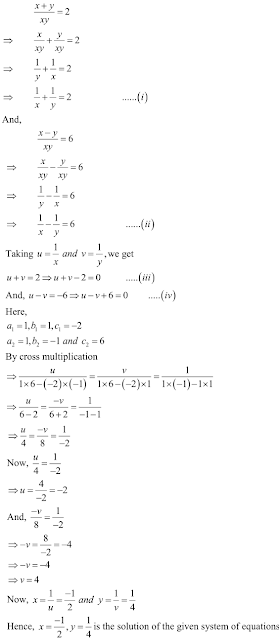6. ax + by = a - b
bx - ay = a + b

Solution

The given system of equations isHence, x = 1, y = 1 is the solution of the given system of the equations.

7. x + ay - b = 0
ax - by - c = 0

Solution

The given system of equations may be written as8. ax + by = a2
bx + ay = b2

Solution

The system of the given equations may be written as9. 5/(x+y) - 2/(x-y) = -1
15/(x+y) + 7/(x-y) = 10
where x ≠ 0 and y ≠ 0

Solution10. 2/x + 3/y = 13
5/x - 4/y = -2
where x ≠ 0 and y ≠ 0

Solution

The given system of equation is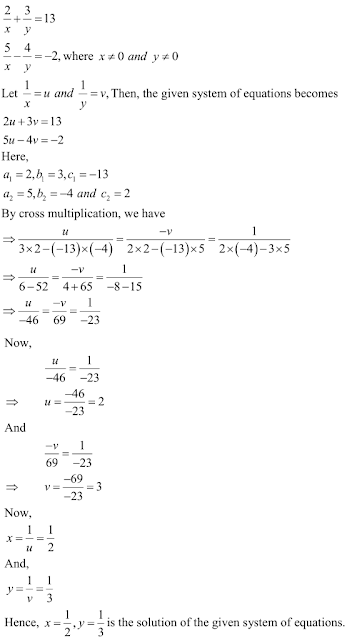11. 57/(x+y) + 6/(x-y) = 5
38/(x+y) + 21/(x-y) = 9

SolutionNow adding eq.(3) and (4) we get x = 11
And after substituting the value of x in eq. (4) we get y = 8
Hence we get the value of x = 11 and y = 8 .

12. x/a + y/b = 2
ax-by = a2 - b2

Solution

The system of the given equations may be written as .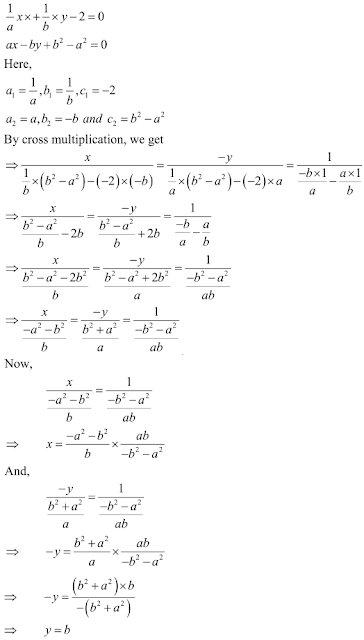13. x/a + y/b = a+b
x/a2 + y/b2 = 2

Solution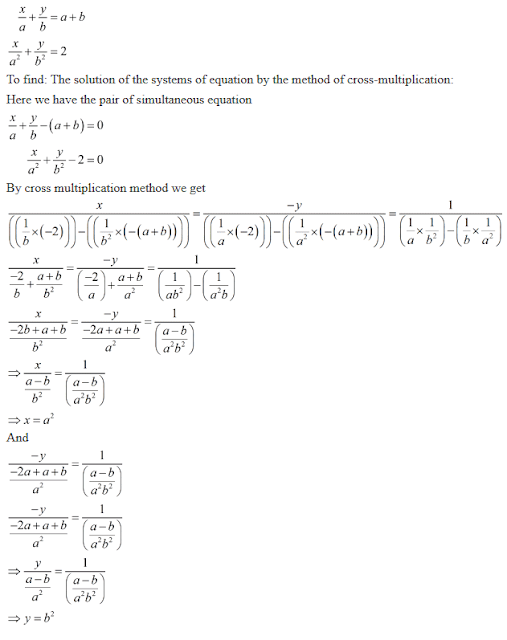Hence we get the value = a2 and y = b2

14. x/a = y/b
ax + by = a2 + b2

Solution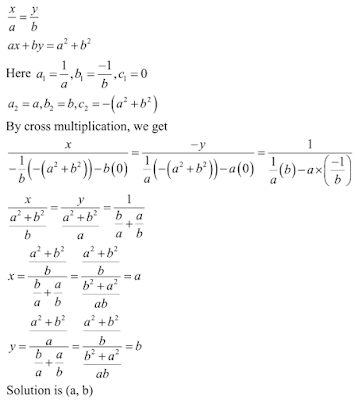15. 2ax + 3by = a + 2b
3ax + 2by = 2a + b

Solution

The given system of equations is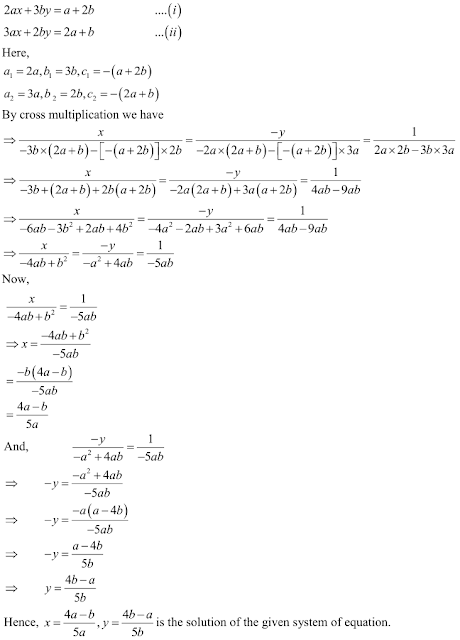16. 5ax + 6by = 28
3ax + 4by - 18 = 0

Solution

The given system of equation is17. (a+2b)x + (2a-b)y = 2
(a-2b)x + (2a+b)y = 3

Solution

The given system of equations may be written as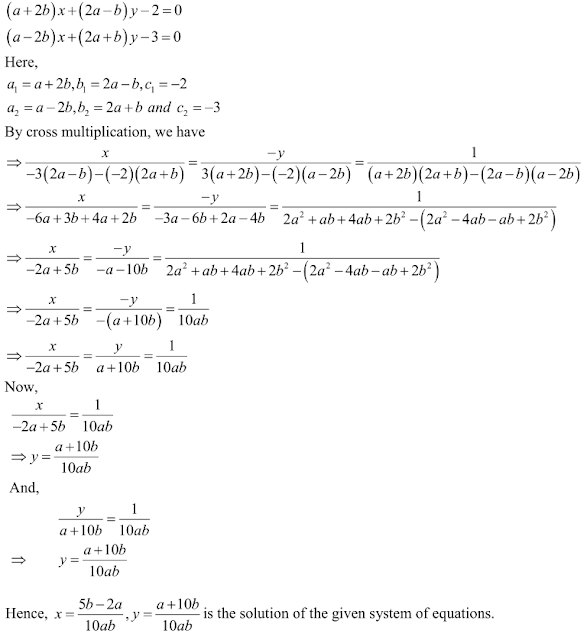18. x[a-b + ab/(a-b)] = y[a+b - ab/(a+b)]
x+y = 2a2

Solution

The given system of equation is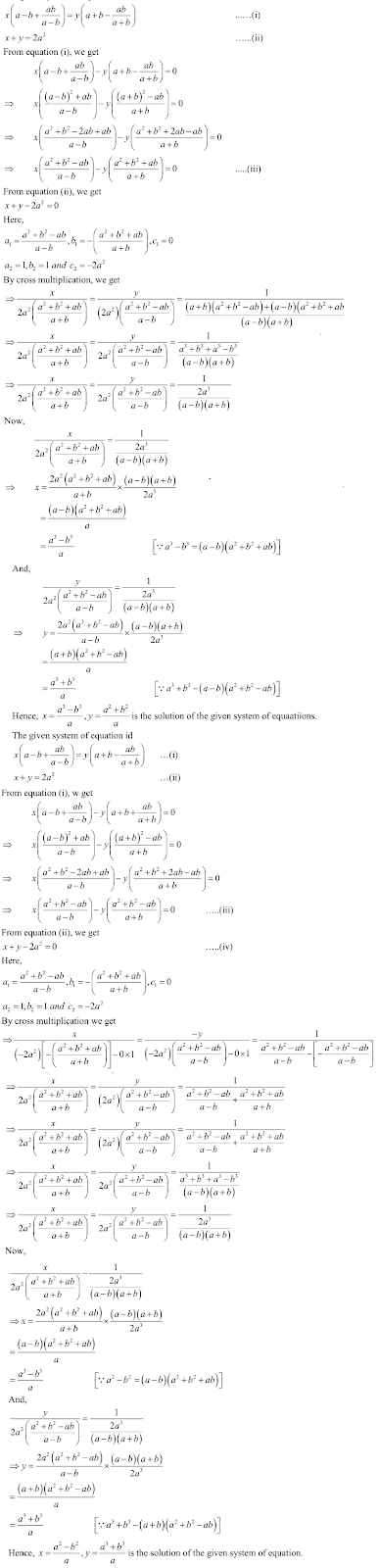19. bx + cy = a + b
ax[1/(a-b) - 1/(a+b)] + cy[1/(b-a) - 1/(b+a) = 2a/(a+b)

Solution

The given system of equation is20. (a-b)x + (a+b)y = 2a2 - 2a2
(a+b) (x+y) = 4ab

Solution

The given system of equation is21. a2x + a2y = c2
b2x + a2y = d2

Solution

The given system of equations may be written as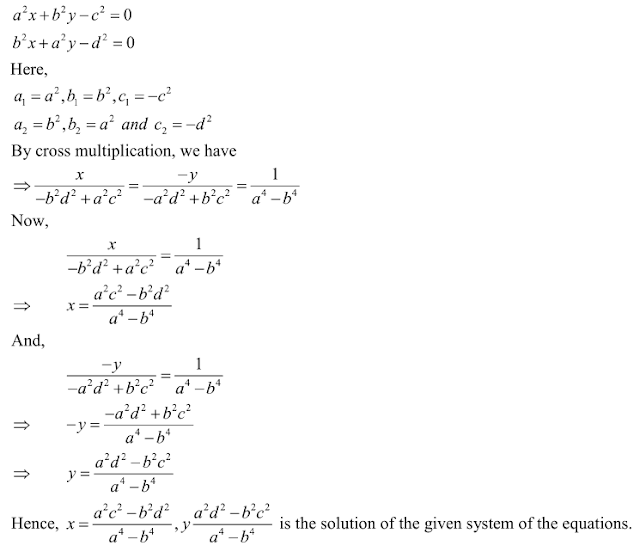22. 57/(x+y) + 6/(x-y) = 5
38/(x+y) + 21/(x-y) = 9

Solution23. 2(ax-by) + a + 4b = 0
2(bx+ay) + b - 4a = 0

Solution

The given system of equation may be written as24. 6(ax + by) = 3a + 2b
6(bx - ay) = 3b - 2a

Solution25. a2/x - b2/y = 0
a2b/x + c2a/y = a + b, x,y ≠ 0

Solution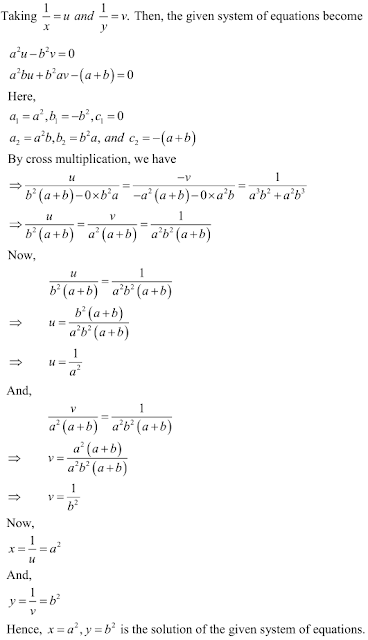26. mx - my = m2 + n2
x + y = 2m

Solution

The given system of equations may be written as27. ax/b - by/a = a+b
ax - by = 2ab

Solution

The given system of equation may be written as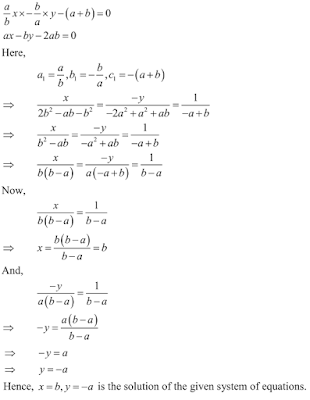28. bx/a + ay/b - (a2 + b2) = 0
x + y - 2ab = 0

Solution

The given system of equation may be written as